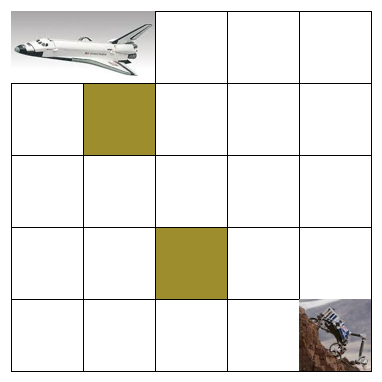Computer Science

Which Algorithm Works WarmupA Nasa robot (bottom right) is trying to rendezvous back with the spaceship (top left). However, it cannot enter the marked yellow squares, which represent unstable areas due to tectonic activity.

Which of these algorithms would guide the robot safely back to the spaceship?

Algorithm 1: Walk 1 square up and 1 square left. Repeat.
Algorithm 2: Walk 2 squares up and 2 squares left. Repeat.
Algorithm 3: Walk 1 square left and 1 square up. Repeat.
Algorithm 4: Walk 2 squares left and 2 squares up. Repeat.

Peter wants to create an algorithm to ensure that he buys exactly N items.

Which of these algorithms could he use?

Algorithm 1: Start with $n =1$.
Buy one item. Increase $n$ by 1.
Repeat. Stop when $n = N$.

Algorithm 2: Start with $n =1$.
Buy one item. Multiply $n$ by 2.
Repeat. Stop when $n = N$.

Algorithm 3: Start with $n =0$.
Buy one item. Increase $n$ by 1.
Repeat. Stop when $n = N$.

Helen wants to create an algorithm that would help her find the largest number in a set. Assume that the set is denoted by a, a, ..., a[N-1].

Which of these algorithms could she use?

Algorithm 1:

• While i < N - 1:
• Set large = a[i].
• Increase i by 1.

Algorithm 2:

• Start with i = 0 and large = a.
• While i < N:
• If a[i] > large, replace large with a[i].
• Increase i by 1.

Algorithm 3:

• Start with i = 0. Set large = a.
• While i < N - 1:
• If a[i] > large, replace large with a[i].
• Increase i by 1.

Jordan wants to create an algorithm to count the number of positive numbers out of an array of real numbers. Assume that the array is denoted by {a, a, ... , a[N - 1]}. Clearly, N is the number of elements in the array a

Which of these algorithms could he use? (They only differ by the first line.)

Algorithm 1:

• Start with i = 0 and total = 0.
• While i < N:
• If a[i] > 0, increase total by 1.
• Increase i by 1.

Algorithm 2:

• Start with i = 0 and total = 1.
• While i < N:
• If a[i] > 0, increase total by 1.
• Increase i by 1.

Algorithm 3:

• Start with i = 1 and total = 0.
• While i < N:
• If a[i] > 0, increase total by 1.
• Increase i by 1.

Susan wants to create an algorithm that finds the Nth Fibonacci number, which follows the rules that

$F_1 = 1, F_2 = 1, F_{n} = F_{n-1} + F_{n-2}.$

Which of the following algorithms could she use to find $F_N$?

Algorithm 1: Set $n=1; \color{#D61F06}{\text{first}} = 1; \color{#3D99F6}{\text{second}} = 1$.
1. If $n = N$, print $\color{#D61F06}{\text{first}}$ and end the program.
2. Set $\color{#D61F06}{\text{first}} = \color{#3D99F6}{\text{second}}; \color{#3D99F6}{\text{second}} = \color{#3D99F6}{\text{second}} + \color{#D61F06}{\text{first}}$ and increase $n$ by 1.
3. Go back to step one.

Algorithm 2: Set $n=1; \color{#D61F06}{\text{first}} = 1; \color{#3D99F6}{\text{second}} = 1$.
1. If $n = N$, print $\color{#D61F06}{\text{first}}$ and end the program.
2. Set $\color{#3D99F6}{\text{second}} = \color{#3D99F6}{\text{second}} + \color{#D61F06}{\text{first}}; \color{#D61F06}{\text{first}} = \color{#3D99F6}{\text{second}}$ and increase $n$ by 1.
3. Go back to step one.

Algorithm 3: Set $n=1; \color{#D61F06}{\text{first}} = 1; \color{#3D99F6}{\text{second}} = 1$.
1. If $n = N$, print $\color{#D61F06}{\text{first}}$ and end the program.
2. Set $\color{#3D99F6}{\text{second}} = \color{#3D99F6}{\text{second}} + \color{#D61F06}{\text{first}}, \color{#D61F06}{\text{first}} = \color{#3D99F6}{\text{second}} - \color{#D61F06}{\text{first}}$ and increase $n$ by 1.
3. Go back to step one.

×